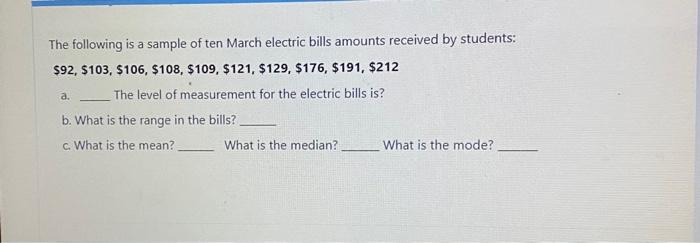# Question please help!Qm exonomics The following is a sample of ten March electric bills amounts received by students: $92,$103, $106,$108, $109,$121, $129,$176, $191,$212 The level of measurement for the electric bills is? b. What is the range in the bills? c. What is the mean? What is the median? What is the mode? a.BFUUQE The Asker · Probability and StatisticsTranscribed Image Text: The following is a sample of ten March electric bills amounts received by students: $92,$103, $106,$108, $109,$121, $129,$176, $191,$212 The level of measurement for the electric bills is? b. What is the range in the bills? c. What is the mean? What is the median? What is the mode? a.
Transcribed Image Text: The following is a sample of ten March electric bills amounts received by students: $92,$103, $106,$108, $109,$121, $129,$176, $191,$212 The level of measurement for the electric bills is? b. What is the range in the bills? c. What is the mean? What is the median? What is the mode? a.